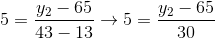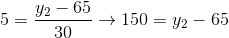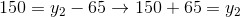# GED Math : Points and Lines

## Example Questions

2 Next →

### Example Question #11 : Points And Lines

Given the points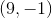and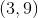, what is the equation of the line?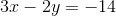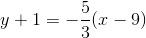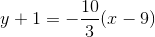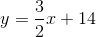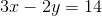Explanation:

The equation of the line is defined in the following forms:

Point-slope form: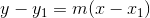Standard form: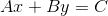Slope intercept form:Find the slope of the two points using the slope formula.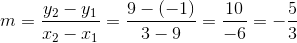Using the slope and any pointor, we can substitute either into the point-slope form.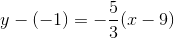The answer is:### Example Question #12 : Points And Lines

Find the slope given the two points: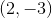and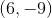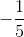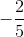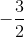Explanation:

Write the slope formula.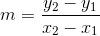Substitute the points.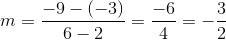The answer is:### Example Question #13 : Points And Lines

Which of the following points is on the line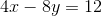?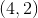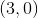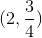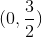Explanation:

In order for a point to be on the line, the point must satisfy the equation given. Thus, plug in theandcoordinates to see if they will give you a true equation.

If you plug ininto the equation, you will get the following: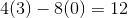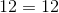Thus,satisfies the equation and must be on the line.

### Example Question #141 : Linear Algebra

Which of the following points is on the following line?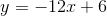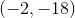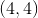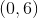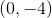Explanation:

Which of the following points is on the following line?So, to test this, we can plug in each choice and solve to see if they make sense.

To save time, let's test the easier ones first. Recall that anything times 0 is 0, so we should try out the options with 0's first.

Recall that ordered pairs represent an x and a y value with the x coming first: (x,y)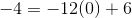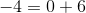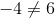So, this is not our answer. However, it does give us a hint as to the correct answer.

When we plugged in 0 for x, we got 6 on the right hand side. This means that if we plug in 0 for x, then we should get 6 for y. So, let's try out our next point.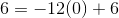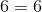So, our answer must be (0,6)

### Example Question #15 : Points And Lines

Which of the following points lies on the line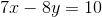?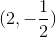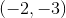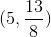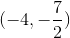Explanation:

In order for a point to be on a line, the point must satisfy the equation. Plug in the values ofandfrom the answer choices to see which one satisfies the equation.

Plugging inwill give the following: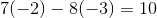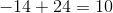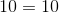Sincesatisfies the given equation, it must be on the line.

### Example Question #16 : Points And Lines

Find the y-coordinate which would would make the slope between the following points equal to 5.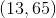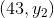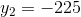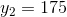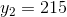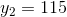Explanation:

Find the y-coordinate which would would make the slope between the following points equal to 5.To find the slope of a line, use the following formula:Now, we know all of these values except one. Let's find it.